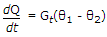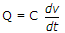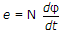# Electronics and Communication Engineering - Automatic Control Systems - Discussion

42.

For the equationwhere Q is heat energy Gt is thermal conductance and θ is temperature, analogous electrical equation is

 [A]. i = G(v1 - v2) [B].[C].[D].Explanation:

Temperatures θ1 and θ2 are analogous to voltage v1 and v2 .